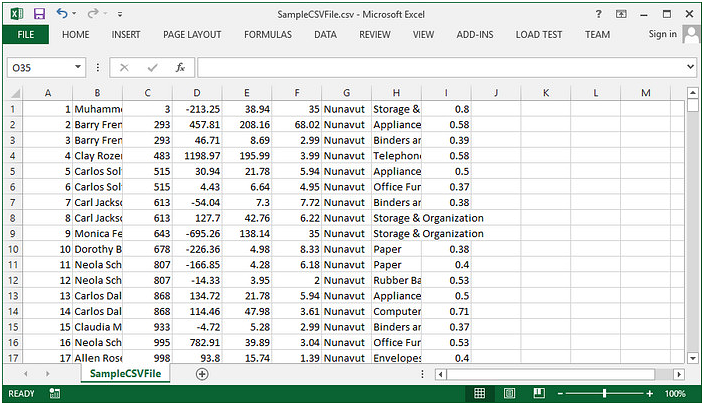Tuesday, 10 October 2017 08:46

## How to Convert CSV to PDF in C#, VB.NET

Written by  support iceblue
Rate this item

Spire.XLS supports to load a CSV file and save as an Excel or PDF file. This tutorial shows how to convert CSV to PDF using Spire.XLS with C# and VB.NET.

The sample CSV file looks as follows.Step 1: Create a Workbook instance and load a sample CSV file.

```Workbook wb = new Workbook();
```

Step 2: To render content of a worksheet into a single PDF page, set the SheetFitToPage property as true.

```wb.ConverterSetting.SheetFitToPage = true;
```

Step 3: Autofit a column if the characters in the column exceed column width.

```Worksheet sheet = wb.Worksheets;
for (int i = 1; i < sheet.Columns.Length; i++)
{
sheet.AutoFitColumn(i);
}
```

Step 4: Save the specified worksheet to PDF.

```sheet.SaveToPdf("toPDF.pdf");
```

Output:Full Code:

[C#]
```Workbook wb = new Workbook();
wb.ConverterSetting.SheetFitToPage = true;
Worksheet sheet = wb.Worksheets;
for (int i = 1; i < sheet.Columns.Length; i++)
{
sheet.AutoFitColumn(i);
}
sheet.SaveToPdf("toPDF.pdf");
```
[VB.NET]
```Dim wb As New Workbook()
wb.ConverterSetting.SheetFitToPage = True
Dim sheet As Worksheet = wb.Worksheets(0)
For i As Integer = 1 To sheet.Columns.Length - 1
sheet.AutoFitColumn(i)
Next
sheet.SaveToPdf("toPDF.pdf")
```